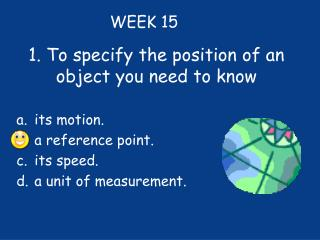DownloadDownload Presentation1. To specify the position of an object you need to know

# 1. To specify the position of an object you need to know

Download Presentation## 1. To specify the position of an object you need to know

- - - - - - - - - - - - - - - - - - - - - - - - - - - E N D - - - - - - - - - - - - - - - - - - - - - - - - - - -
##### Presentation Transcript

1. 1. To specify the position of an object you need to know WEEK 15 • its motion. • a reference point. • its speed. • aunit of measurement.

2. 2. When something changes position, what is it doing? • It is static. • It is a reference point. • It is still. • It is moving.

3. 3. Mass is • different on the moon than on Earth. • a measure of gravitational force. • a measure of the amount of matter. • measured in Newtons.

4. 4. A ball rolls a distance of 1 meter for 2 seconds. What is the speed of the ball? • 2 m/s • 1.5 m/s • 1 m/s • 0.5 m/s

5. 5. The difference between speed and velocity is that http://www.youtube.com/watch?v=DRb5PSxJerM • velocity involves time, while speed does not. • speed involves time, while velocity does not. • velocity has direction, while speed does not. • speed has direction, while velocity does not.

6. 6. Which tell you how quickly an object changes position? • Direction • Relative motion • Speed • observation

7. 7. A bug travels fast and then slows down. It stops for 10 seconds. It then walks slowly, speeding up as it goes. Which graph shows the bug’s speed? a. c. d. b.

8. 8. If you measure the total straight line distance from an object’s starting point to its ending point, you are measuring • distance • displacement • speed • velocity

9. 9. A hiker’s velocity begins at 1.8 m/s uphill and changes to 1.5 m/s uphill. How do you know that the hiker has a negative acceleration? • her direction changed • her direction did not change • her speed increased • her speed decreased

10. 10. Which of these is a unit of acceleration? • m/s • h • km • m/s2